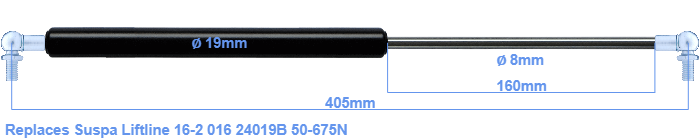# Replacement for Suspa Liftline 16-2 016 24019B 50-675N

\$40.11

Replacement gas spring for the Suspa Liftline 16-2 016 24019B 50-675 Newton. This fits a (possibly already present) ball with a diameter of 10mm. A corresponding ball is included. Brand: Stabilus Industry Line.
 Force Choose an option50 Newton60 Newton80 Newton100 Newton120 Newton140 Newton150 Newton160 Newton180 Newton200 Newton220 Newton240 Newton250 Newton260 Newton280 Newton300 Newton320 Newton340 Newton350 Newton360 Newton380 Newton400 Newton420 Newton440 Newton450 Newton460 Newton480 Newton500 Newton520 Newton540 Newton550 Newton560 Newton580 Newton600 Newton620 Newton640 Newton650 Newton660 Newton675 NewtonClearThe diameter of the cylinder of this gas spring is 19 millimeters. The cross section of the rod is 8 mm. The stroke (the retracting part) has a length of 160 mm. The total length of this replacement is 405 millimeter. Note: this is the total length between the rotation points of the two mounting parts. Without the mounting parts this gas spring is 365 mm long (thread to thread). This replacement gas spring has a force of 50-675 Newton. This is not an official Suspa Liftline gas spring, but a so called replacement. This is a Stabilus Industry Line gas spring. The force (50-675 N) and dimensions are identical to the original.
Category: